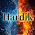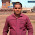## Electronics Devices Objective Type Questions

 A Zener diode is used for
a) Voltage Regulation
b) Rectification
c) Noise Suppression
d) Blocking A.C

 An SCR is a device having
a) Three layers with four junctions
b) Three layers with two junctions
c) Four layers with three junctions
d) Two layers with three junctions

 An amplifier has a gain of 10,000 expressed in decibels the gain is
a) 10
b) 40
c) 80
d) 100

 An emitter follows has
a) High input impedance and high output impedance.
b) High input impedance and low output impedance.
c) Low input impedance and high output impedance.
d) Low input impedance and low output impedance.

 Semi-conductor diode time constant is equal to
a) The value of majority carrier life time
b) The life time of minority carrier
c) The diffusion capacitance time constant
d) Zero

 To prepare a P type semiconducting material the impurities to be added to silicon are
a) Boron, Gallium
b) Arsenic, Antimony
c) Gallium, Phosphorous
d) Gallium, Arsenic

 FET is a good signal chopper because
a) It exhibits no offset voltage at zero drain current
b) It occupies less space in integrated form
c) It is less noisy
d) It has got high input impedance

 In Bipolar Junction transistors, the type of configuration which will give both voltage gain and current gain is
a) CC
b) CB
c) CE
d) None

 To increase the input resistance and decrease the output resistance in negative feedback, the type used is
a) Voltage Shunt
b) Current Series
c) Voltage Series
d) Current Shunt

 A series capacitance used in a filter circuit represents
a) Low-Pass
b) Band-Pass
c) High-Pass
d) None

 An ideal power supply is characterized by
a) Very large output resistance
b) Very small output resistance
c) Zero internal resistance
d) Infinite internal resistance

 An ideal diode should have
a) Zero resistance in the forward bias as well as reverse bias
b) Zero resistance in the forward bias and an infinitely large resistance in reverse bias
c) Infinitely large resistance in reverse bias
d) Infinitely large resistance in forward as well as reverse bias

 One coulomb-per-second is equal to one:
a) Watt
b) Joule
c) Volt
d) Ampere

 Which of the following is one of the functions performed by a diode?
a) Filter
b) Amplifier
c) Rectifier
d) Inverter

 What is the “power factor”?
a) Ratio of true power to apparent power
b) Peak power times 0.707
c) Sin of the phase difference between E and I
d) Cos of the phase angle between true power and apparent power

Solution Hint:
 General purpose diode is used for rectification, whereas zener diode is for voltage regulation
 Power gain = 10000
Gain in dB = 10log(10000) = 40dB
Voltage gain = 10000
Gain in dB = 20log(10000) = 80dB
 In the Emitter follower circuit, the input side (base-collector terminals) is reverse biased, ie, High input impedance and the Output side (emitter-collector terminals) is forward biased ie, low output impedance
 Ideal power supply should not dissipate heat ( ie, internal resistance should be near to zero)
 Ideal diode is nothing but ideal DC switch( zero resistance while turned-on and infinity resistance while turned-off)
 Flow of charges (unit = coulomb) is known as current(unit = ampere).
9:42 AM

#### 6 comments:

1.How the gain of 10000 expressed in decibels is 100...plz explain

2.3.wrong answer of question (3) gain of 10000 = 100 ??

4.answer of question 3 is wrong gain of 10000= 100?

5.6.power gain = 10000
Gain in dB = 10log(10000) = 40dB
voltage gain = 10000
Gain in dB = 20log(10000) = 80dB

So where did 100 come from?